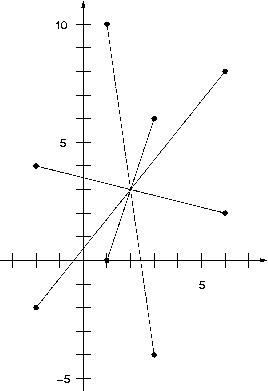문제1868--Center of symmetry

### 1868: Center of symmetry

실행시간 제한: 1 Sec  메모리사용 제한: 64 MB
제출: 61  통과: 13
[제출] [채점기록] [묻고답하기]

#### 문제 설명Given is a set of n points with integer coordinates. Your task is to decide whether the set has a center of symmetry.

A set of points $S$ has the center of symmetry if there exists a point s (not necessarily in $S$) such that for every point $p$ in $S$ there exists a point $q$ in $S$ such that $p-s = s-q$.

#### 입력 설명

The first line of input contains a number $c$ giving the number of cases that follow. The first line of data for a single case contains number $1 \leq n \leq 10000$. The subsequent $n$ lines contain two integer numbers each which are the $x$ and $y$ coordinates of a point. Every point is unique and we have that $-10000000 \leq x, y \leq 10000000$.

#### 출력 설명

For each set of input data print yes if the set of points has a center of symmetry and no otherwise.

#### 입력 예시 Copy

1
8
1 10
3 6
6 8
6 2
3 -4
1 0
-2 -2
-2 4


#### 출력 예시 Copy

yes